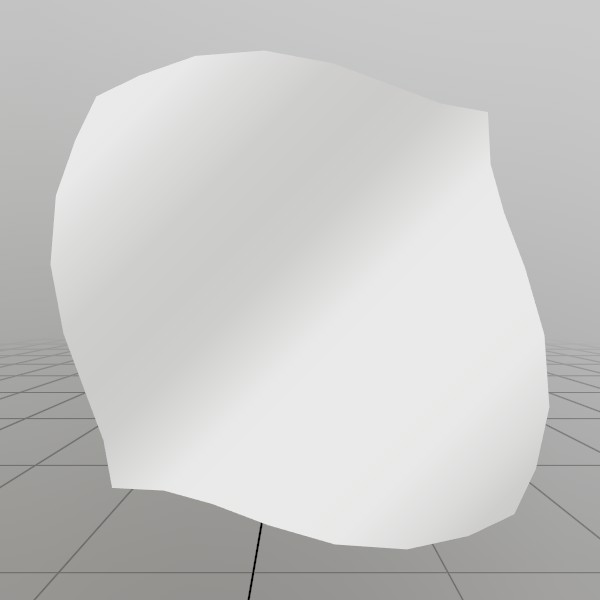# Mesh.SetInds

``````void SetInds(UInt32[] inds)
``````

Assigns the face indices for this Mesh! Faces are always triangles, there are only ever three indices per face. This function will create a index buffer object on the graphics card right away. If you’re calling this a second time, the buffer will be marked as dynamic and re-allocated. If you’re calling this a third time, the buffer will only re-allocate if the buffer is too small, otherwise it just copies in the data!

UInt32[] inds A list of face indices, must be a multiple of 3. Each index represents a vertex from the array assigned using SetVerts.

## Examples

### Procedurally generating a wavy gridHere, we’ll generate a grid mesh using Mesh.SetVerts and Mesh.SetInds! This is a common example of creating a grid using code, we’re using a sin wave to make it more visually interesting, but you could also substitute this for something like sampling a heightmap, or a more interesting mathematical formula!

Note: x+y*gridSize is the formula for 2D (x,y) access of a 1D array that represents a grid.

``````const int   gridSize = 8;
const float gridMaxF = gridSize-1;
Vertex[] verts = new Vertex[gridSize*gridSize];
uint  [] inds  = new uint  [gridSize*gridSize*6];

for (int y = 0; y < gridSize; y++) {
for (int x = 0; x < gridSize; x++) {
// Create a vertex on a grid, centered about the origin. The dimensions extends
// from -0.5 to +0.5 on the X and Z axes. The Y value is then sampled from
// a sin wave using the x and y values.
//
// The normal of the vertex is then calculated from the derivative of the Y
// value!
verts[x+y*gridSize] = new Vertex(
new Vec3(
x/gridMaxF-0.5f,
SKMath.Sin((x+y) * 0.7f)*0.1f,
y/gridMaxF-0.5f),
new Vec3(
-SKMath.Cos((x+y) * 0.7f),
1,
-SKMath.Cos((x+y) * 0.7f)).Normalized,
new Vec2(
x / gridMaxF,
y / gridMaxF));

// Create triangle face indices from the current vertex, and the vertices
// on the next row and column! Since there is no 'next' row and column on
// the last row and column, we guard this with a check for size-1.
if (x<gridSize-1 && y<gridSize-1)
{
int ind = (x+y*gridSize)*6;
inds[ind  ] = (uint)((x+1)+(y+1)*gridSize);
inds[ind+1] = (uint)((x+1)+(y  )*gridSize);
inds[ind+2] = (uint)((x  )+(y+1)*gridSize);

inds[ind+3] = (uint)((x  )+(y+1)*gridSize);
inds[ind+4] = (uint)((x+1)+(y  )*gridSize);
inds[ind+5] = (uint)((x  )+(y  )*gridSize);
}
} }
demoProcMesh = new Mesh();
demoProcMesh.SetVerts(verts);
demoProcMesh.SetInds (inds);
``````

Found an issue with these docs, or have some additional questions? Create an Issue on Github!